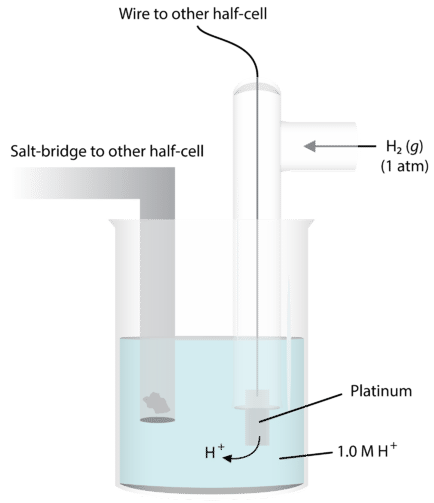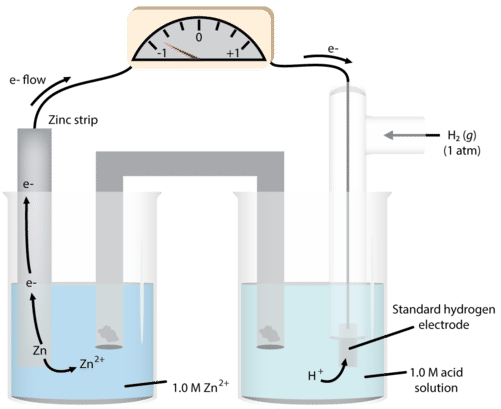23.5: Standard Hydrogen Electrode

We all compare ourselves to someone. Can I run faster than you? Am I taller than my dad? These are relative comparisons that don't give a lot of useful data. When we use a standard for our comparisons, everybody can tell how one thing compares to another. One meter is the same distance everywhere in the world, so a 100 meter track in one country is exactly the same distance as a 100 meter track in another country. We now have a universal basis for comparison.

Standard Hydrogen Electrode

The activity series allows us to predict the relative reactivities of different materials when used in oxidation-reduction processes. We also know we can create electric current by a combination of chemical processes. But how do we predict the expected amount of current that will flow through the system? We measure this flow as voltage (an electromotive force or potential difference).

In order to do this, we need some way of comparing the extent of electron flow in the various chemical systems. The best way to do this is to have a baseline that we use - a standard that everything can be measured against. For determination of half-reaction current flows and voltages, we use the standard hydrogen electrode. The figure below illustrates this electrode. A platinum wire conducts the electricity through the circuit. The wire is immersed in a $$1.0 \: \text{M}$$ strong acid solution and $$\ce{H_2}$$ gas is bubbled in at a pressure of one atmosphere and a temperature of $$25^\text{o} \text{C}$$. The half-reaction at this electrode is $$\ce{H_2} \rightarrow 2 \ce{H^+} + 2 \ce{e^-}$$.Figure 23.5.1: The standard hydrogen electrode.

Under these conditions, the potential for the hydrogen reduction is defined as exactly zero. We call this $$E^0$$, the standard reduction potential.

We can then use this system to measure the potentials of other electrodes in the half-cell. A metal and one of its salts (sulfate is often used) is in the second half-cell. We will use zinc as our example (see figure below).Figure 23.5.2: The standard hydrogen half-cell paired with a zinc half-cell.

As we observe the reaction, we notice that the mass of solid zinc decreases during the course of the reaction. This suggests that the reaction occurring in that half-cell is

$\ce{Zn} \left( s \right) \rightarrow \ce{Zn^{2+}} \left( aq \right) + 2 \ce{e^-}$

So, we have the following process occurring in the cell:

\begin{align} &\ce{Zn} \left( s \right) \rightarrow \ce{Zn^{2+}} \left( aq \right) + 2 \ce{e^-} \: \left( \text{anode - oxidation} \right) \\ &2 \ce{H^+} \left( aq \right) + 2 \ce{e^-} \rightarrow \ce{H_2} \left( g \right) \: \left( \text{cathode - reduction} \right) \end{align}

and the measured cell voltage is 0.76 volts (abbreviated as $$\text{V}$$.

We define the standard emf (electromotive force) of the cell as:

\begin{align} E^0_\text{cell} &= E^0_\text{cathode} - E^0_\text{anode} \\ 0.76 \: \text{V} &= 0 - E^0_{\ce{Zn} \: \text{cell}} \\ E^0_{\ce{Zn} \: \text{cell}} &= -0.76 \: \text{V} \end{align}

We can do the same determination with a copper cell (figure below).Figure 23.5.3: The standard hydrogen half-cell paired with a copper half-cell.

as we run the reaction, we see the mass of the copper increases, so we write the half-reaction:

$\ce{Cu^{2+}} + 2 \ce{e^-} \rightarrow \ce{Cu}$

This makes the copper electrode the cathode. We now have the two half-reactions:

\begin{align} &\ce{H_2} \rightarrow 2 \ce{H^+} + 2 \ce{e^-} \: \left( \text{anode} \right) \\ &\ce{Cu^{2+}} + 2 \ce{e^-} \rightarrow \ce{Cu} \: \left( \text{cathode} \right) \end{align}

and we determine the $$E^0$$ for the system to be $$0.34 \: \text{V}$$.

Again,

\begin{align} E^0_\text{cell} &= E^0_\text{cathode} - E^0_\text{anode} \\ 0.34 \: \text{V} &= E^0_\text{copper} - 0 \end{align}

so copper potential $$= 0.34 \: \text{V}$$.

Now we want to build a system in which both zinc and copper are involved. We know from the activity series that zinc will be oxidized and copper reduced, so we can use the values at hand:

$E^0_\text{cell} = 0.34 \: \text{V} \: \left( \text{copper} \right) - \left( -0.76 \: \text{V} \: \text{zinc} \right) = 1.10 \: \text{volts for the cell}$

Summary

• The structure of the standard hydrogen electrode is described.
• Examples of using this electrode to determine reduction potentials are given.

Contributors

• CK-12 Foundation by Sharon Bewick, Richard Parsons, Therese Forsythe, Shonna Robinson, and Jean Dupon.